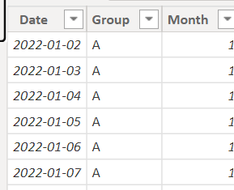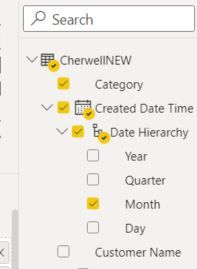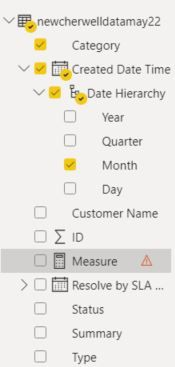cancel
Showing results for
Did you mean:Helper II

## Report Help -

Hi

Im trying to Create a report that lists by month, top 10 categories month by month, some will change each months so in need to reflect this , is this possible?1 ACCEPTED SOLUTIONCommunity Support

Hi  @dommyw277 ,

I created some data:Here are the steps you can follow：

1. Create measure.

``````Count =
var _select=SELECTEDVALUE('Table'[Month])
return
COUNTX(FILTER(ALL('Table'),'Table'[Month]=_select&&'Table'[Group]=MAX('Table'[Group])),[Group])``````
``````rank =
RANKX(ALL('Table'),[Count],,DESC,Dense)``````
``````Flag =
IF(
[rank]<=10,1,0)``````

2. Place [Flag]in Filters, set is=1, apply filter.3. Result:For errors, you can refer to:

https://community.powerbi.com/t5/Desktop/Column-cannot-be-found-or-may-not-be-used-in-this-expressio...

Best Regards,

Liu Yang

If this post helps, then please consider Accept it as the solution to help the other members find it more quickly

11 REPLIES 11Community Support

Hi  @dommyw277 ,

I created some data:Here are the steps you can follow：

1. Create measure.

``````Count =
var _select=SELECTEDVALUE('Table'[Month])
return
COUNTX(FILTER(ALL('Table'),'Table'[Month]=_select&&'Table'[Group]=MAX('Table'[Group])),[Group])``````
``````rank =
RANKX(ALL('Table'),[Count],,DESC,Dense)``````
``````Flag =
IF(
[rank]<=10,1,0)``````

2. Place [Flag]in Filters, set is=1, apply filter.3. Result:For errors, you can refer to:

https://community.powerbi.com/t5/Desktop/Column-cannot-be-found-or-may-not-be-used-in-this-expressio...

Best Regards,

Liu Yang

If this post helps, then please consider Accept it as the solution to help the other members find it more quicklyHelper II

Thank you so much! Works a treatHelper II

So using the table I have called ReportData , the fields are Category and Month , I have this - can anyone help where im going wrong please?

calculate(Sum(ReportData[Category]) ,TOPN(10,all(ReportData[Category]),calculate(Sum(ReportData[Month]) )), values(ReportData[Category]))Super User

Hi,

This measure is correct.  What problem are you facing?

Regards,
Ashish Mathur
http://www.ashishmathur.comHelper II

The issue appears to be that im trying to SUM Text fields so maybe thats why the TOP n argument isnt working?

calculate(Sum(ReportData[Category]) ,TOPN(10,all(ReportData[Category]),calculate(Sum(ReportData[Month]) )), values(ReportData[Category]))Super User

Hi,

Does this work?

=calculate(countrows(ReportData) ,TOPN(10,all(ReportData[Category]),calculate(Sum(ReportData[Month]) )), values(ReportData[Category]))

Regards,
Ashish Mathur
http://www.ashishmathur.comHelper II

Hi, so ReportData is replaced by NewCherwell however i get these messages. Maybe because Month is a part of created date time?Super User

Hi,

Regards,
Ashish Mathur
http://www.ashishmathur.comHelper II

Hi im getting this message:

ReportData is replaced by CherwellNEW for infoHelper IIHi, what do i put where the value is?Super User

@dommyw277 , Create a TOPN measure and display is with month and category

calculate(Sum(Table[Value]) ,TOPN(1,all(Table[category]),calculate(Sum(Table[Value]) )), values(Table[category]))

or refer this

https://www.sqlbi.com/articles/filtering-the-top-3-products-for-each-category-in-power-bi/